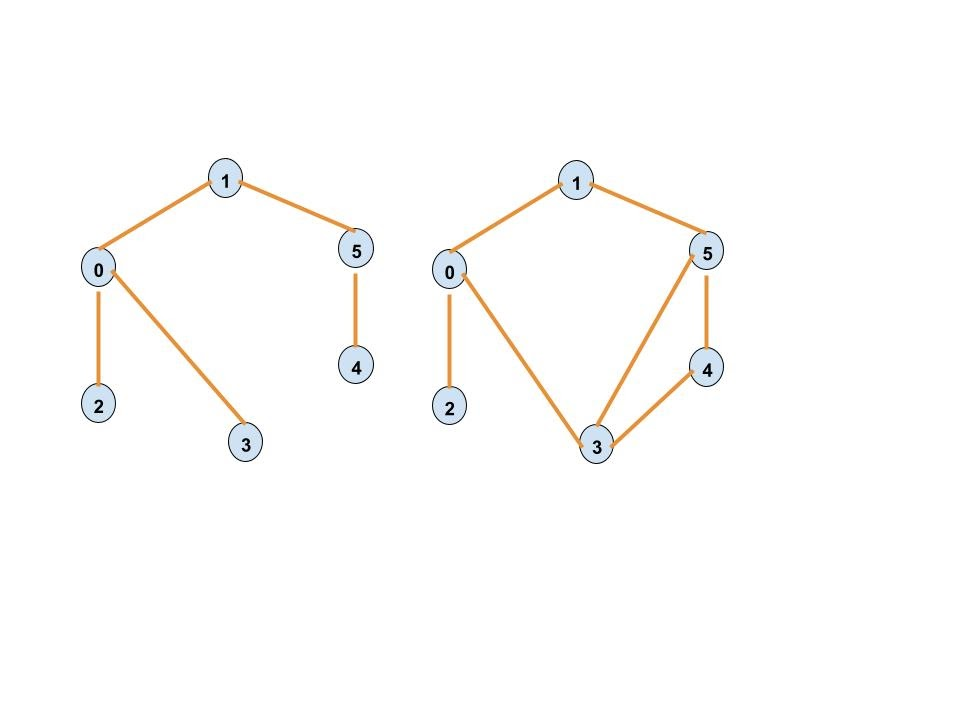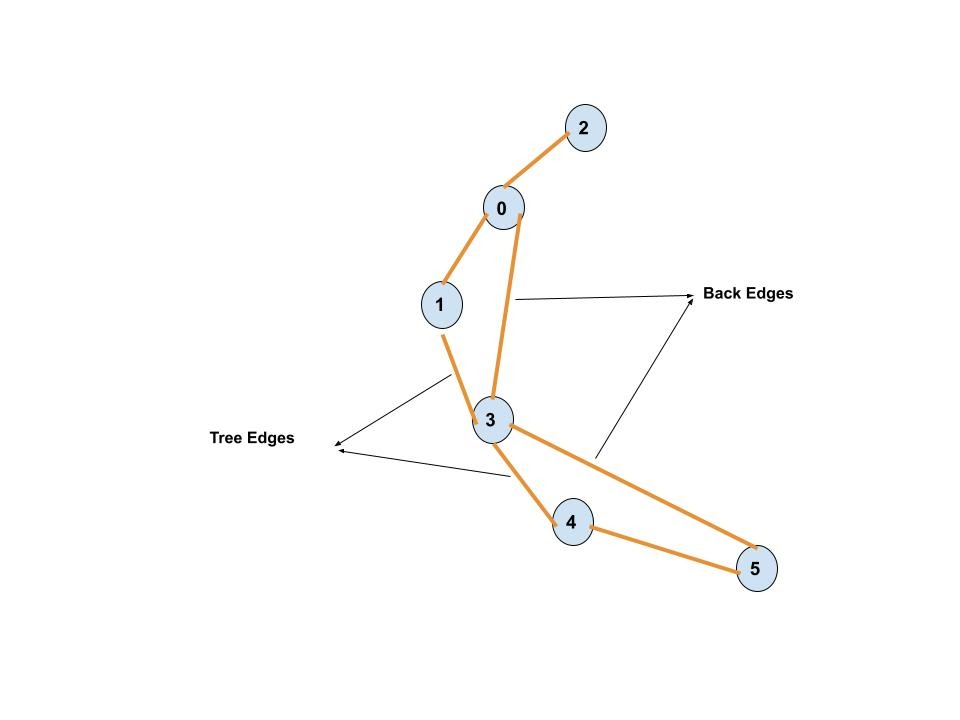# How to detect cycles in an undirected graph

## Introduction

In this blog, we will be discussing a method to detect cycles in an undirected graph. Before diving into the solution, let's again look at what exactly is an undirected graph and a cycle in it.

A graph whose edges have no preference for the direction of data flowing through them is called an undirected graph, and a cycle in a graph is a non-empty trail with repeated first and last vertices.For example, in the above figure, the graph on the right contains a cycle.

## Solution using DFS

This approach is very similar to the method for detecting cycles in a directed graph. In this method also, we use DFS to detect cycles in an undirected graph as the DFS of a graph will produce a DFS tree, which is a reordered representation of the edges and vertices of the graph. In this DFS tree, we have to find a back edge, an edge either connecting a vertex to itself or its ancestor. If we find such an edge, we will conclude that the graph is cyclic.In the above diagram, let us say we start DFS from node 2 and we visit the nodes: 0, 1, 3, 4 and 5 through the edges: 2->0, 0->1, 1->3, 3->4 and 4->5. These edges become the forward edges in our DFS traversal of the graph which eventually form a DFS tree considering the forward edges only whereas the edges which were not the part of our DFS i.e 0->3 and 3->5 become the back edges.

We will be using an array of visited vertices during the DFS traversal to check for back edges, so whenever we find an edge that goes back to a vertex already in the visited array, we return true as a result that the graph contains a cycle.

## Algorithm for the solution

1. Take the number of edges and vertices as user input.
2. Use vertex visited array and parent node to create a recursive function.
3. Mark the current vertex as visited.
4. Make recursive calls for all the unvisited adjacent vertices for the current vertex, and if any of these recursive functions return true, return true as the final answer.
6. Return false if none of the above steps returns true.

## Implementation of the solution

Output-

The time complexity of the above approach to detect cycles in a directed graph is O(V+E), where V is the number of vertices in the graph and E is the number of edges. This happens because we are doing the DFS of the tree, which has the same time complexity.

The space complexity of the above approach is O(V).

Q1. How do you determine if an undirected graph has a cycle?

If an undirected graph has a back edge in its DFS tree, we can conclude that it contains a cycle.

Q2. How many cycles are in a complete graph?

The total number of cycles in a complete graph is (n+1)!.

Q3. Can BFS be used to find cycles in undirected graphs?

We can use BFS for cycle detection in undirected graphs, but it takes more memory than DFS based methods.

Q4. Can a cycle repeat edges?

No, a cycle can not have repeated edges or vertices, as it is a closed path.

Q5. Does a complete graph have a Hamiltonian cycle?

The Hamiltonian cycle in a graph is a closed-loop with every node being visited only once.

## Key takeaways

In this blog, we learned to detect cycles in an undirected graph.

To detect the cycles in an undirected graph, we started with a Depth-first search traversal of the graph. We maintain an array of all the visited vertices, and during the traversal, whenever we get an edge that goes back to an already visited vertex, also known as a back edge. We return the answer that the graph is cyclic.

You must check out the blog on the detecting cycle in a directed graph as well. You can practice similar problems on CodeStudio as well.